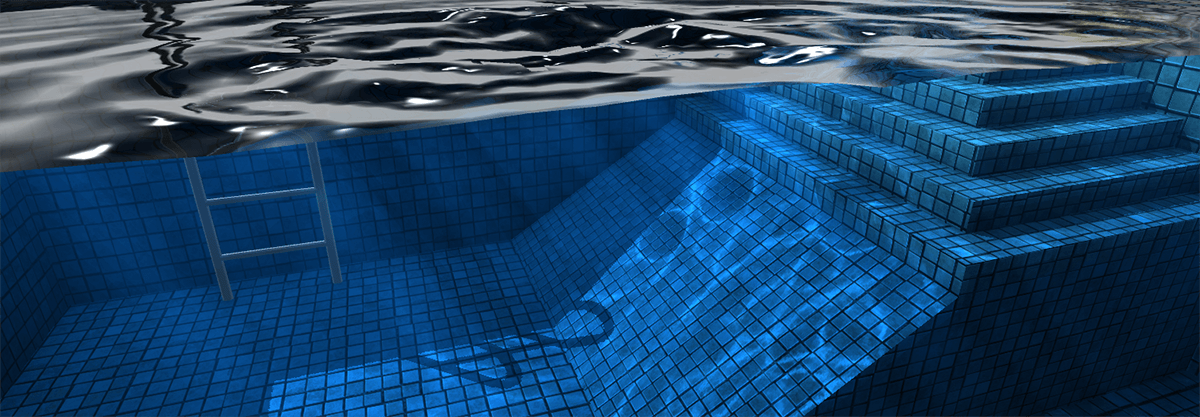# 如何拥有一个“私人泳池”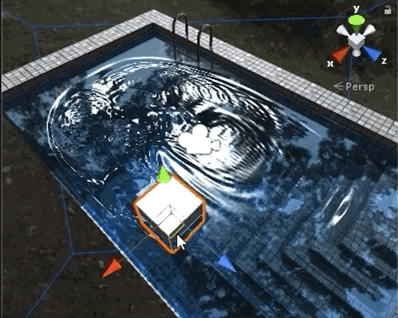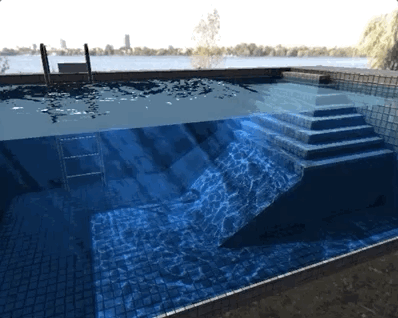## 项目链接

Wave Equation
https://lab.uwa4d.com/lab/5b5a5467d7f10a201fe4bbfb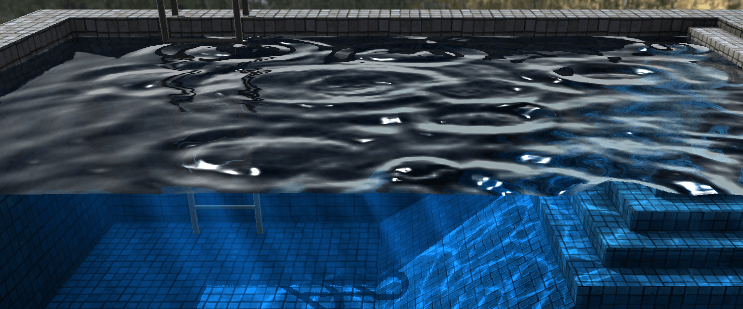LiquidSimulator.cs用于控制生成水面网格，项目运行后会在当前GameObject中添加Mesh组件与水面材质，并添加一个摄像机用于实现反射效果（ReflectCamera）。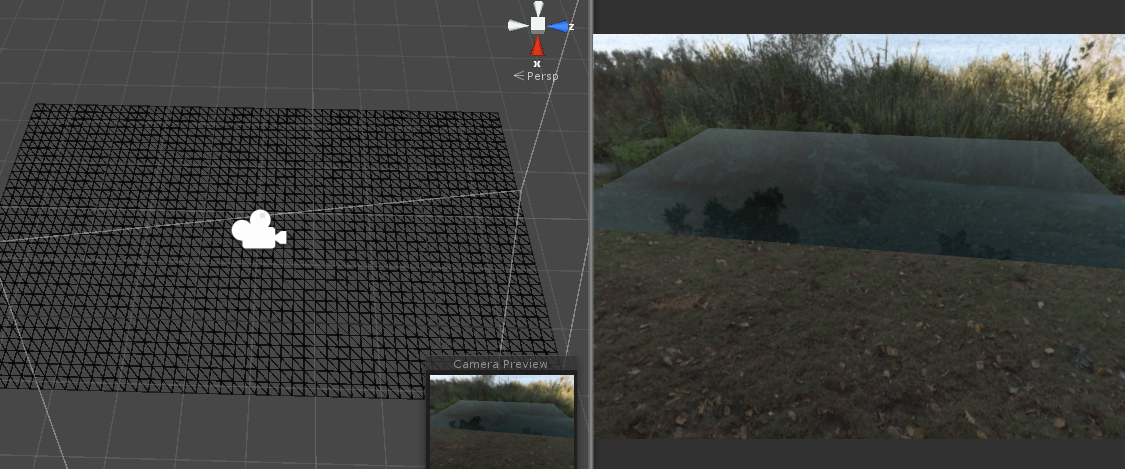LiquidSimulator的Inspector面板中输入参数：水体长度、宽度、深度、网络单元格大小、粘性阻尼大小、波速、力度系数。这些参数在经过波速、时间等约束条件检测后传递给LiquidSampleCamera.cs用于构建摄像机（LiquidSampleCamera）。

LiquidSampleCamera将水面作为近裁截面，生成名为[Cur]的RenderTexture作为当前摄像机的targetTexture。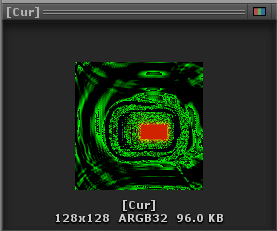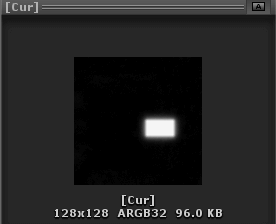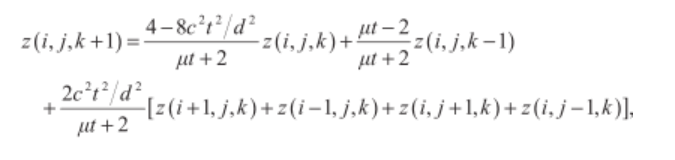[Pre]为上一个时间段的位移RT、[Cur]为当前时间段的位移RT。

`````` 1float cur = _WaveParams.x*DecodeHeight(tex2D(_MainTex, i.uv));
2
3float rg = _WaveParams.z*(
4  DecodeHeight(tex2D(_MainTex, i.uv + float2(_WaveParams.w, 0)))
5  + DecodeHeight(tex2D(_MainTex, i.uv + float2(-_WaveParams.w, 0)))
6  + DecodeHeight(tex2D(_MainTex, i.uv + float2(0, _WaveParams.w)))
7  + DecodeHeight(tex2D(_MainTex, i.uv + float2(0, -_WaveParams.w))));
8
9float pre = _WaveParams.y*DecodeHeight(tex2D(_PreTex, i.uv));
10
11cur += rg + pre;
``````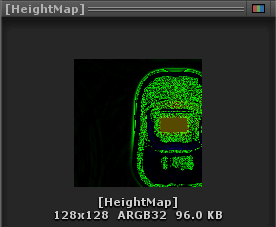[HeightMap]RT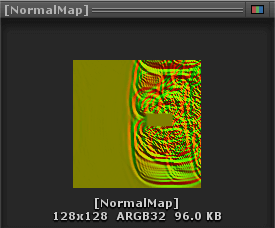[NormalMap]RT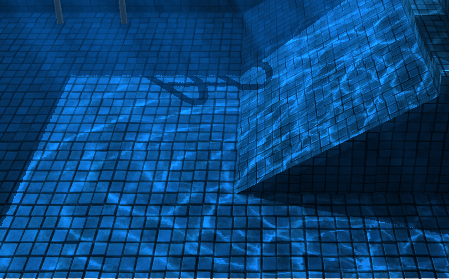CausticRenderer组件执行开始时创建一个摄像机，并新建一个顶点较多的网格。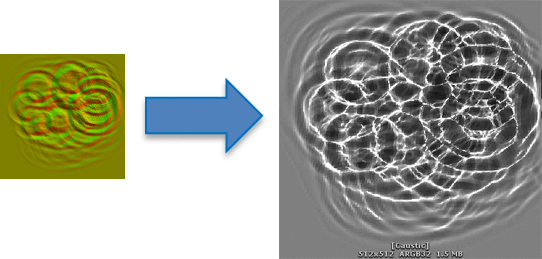`````` 1v2f vert (appdata_full v)
2{
3  v2f o;
4  float3 normal = UnpackNormal(tex2Dlod(_LiquidNormalMap,float4(v.texcoord.xy,0,0)));
5  o.oldPos = v.vertex.xz;
6  v.vertex.xz += normal.xy*_Refract;
7  o.newPos = v.vertex.xz;
8  o.vertex = UnityObjectToClipPos(v.vertex);
9   return o;
10}
``````
``````1fixed4 frag (v2f i) : SV_Target
2{
3  float oldArea = length(ddx(i.oldPos)) * length(ddy(i.oldPos));
4  float newArea = length(ddx(i.newPos)) * length(ddy(i.newPos));
5  //发生折射后与未折射时三角面的面积比
6  float area = (oldArea / newArea) * 0.5;
7  return float4(area, area, area, 1);
8}
``````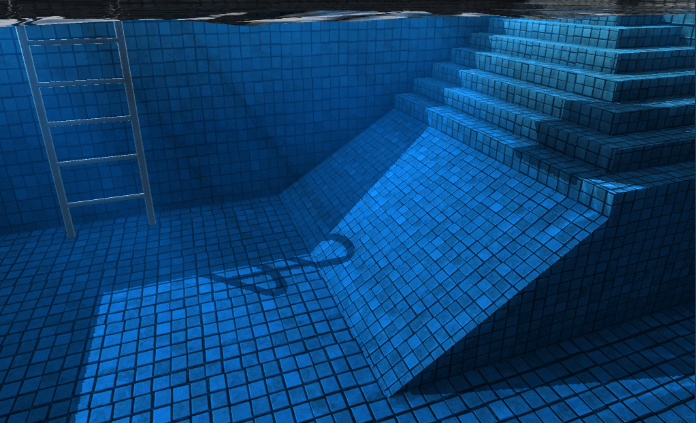`````` 1//传入水体包围盒信息，用于计算水底光线追踪的范围
2Vector3 boundsMin = new Vector3(transform.position.x - width * 0.5f,
3  transform.position.y - depth,
4  transform.position.z - length * 0.5f);
5Vector3 boundsMax = new Vector3(transform.position.x + width * 0.5f,
6  transform.position.y,
7  transform.position.z + length * 0.5f);
8
9//传入水体平面用于获取水体表面法线，以计算折射光线
10Vector4 plane = new Vector4(0, 1, 0, Vector3.Dot(new Vector3(0, 1, 0), transform.position));
``````
`````` 1float3 boundSize = _BoundsMax - _BoundsMin;
2float delta = max(boundSize.x, max(boundSize.y, boundSize.z)) / RAY_STEP;
3float coldelta = 1.0 / RAY_STEP * _LightIntensity;
4float3 viewDir = normalize(-UnityWorldSpaceViewDir(i.worldPos));
5float4 col = float4(0, 0, 0, 0);
6for (float k = 0; k < RAY_STEP; k++) {
7  float3 wp = i.worldPos + viewDir * k * delta;
9  float clipv = ClipInBounds(wp);
10  float ndl = SampleWaterNormalDotLightDir(wp);
11  col += _FilterColor * coldelta * atten * clipv*ndl;
12}
13return col;
``````

1.水面反射效果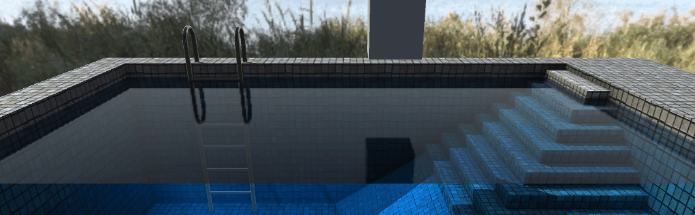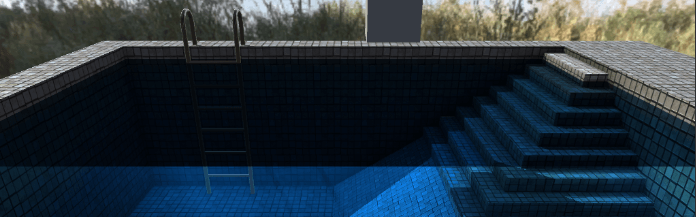2.阴影效果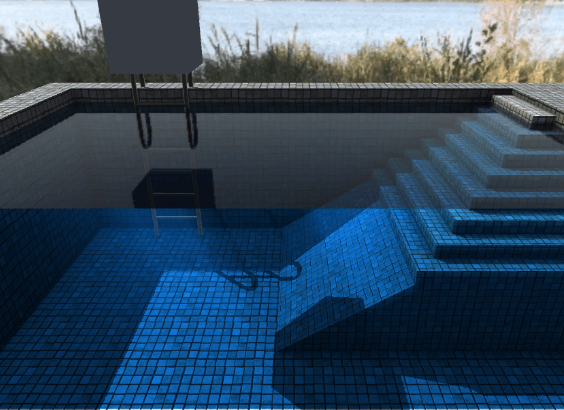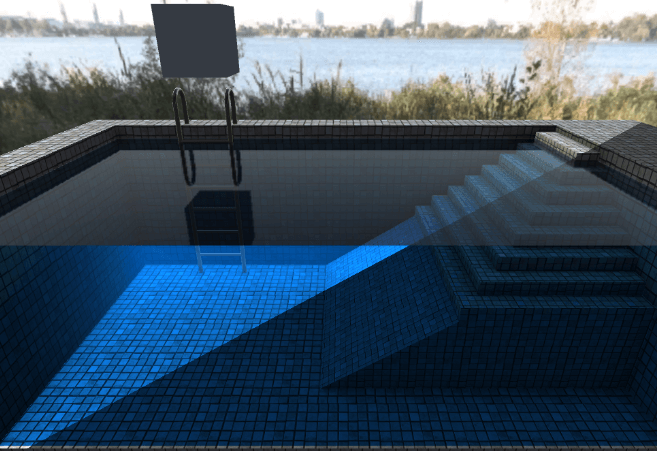3.BlinnPhong光照模型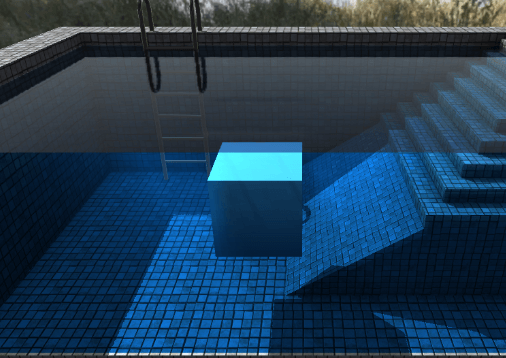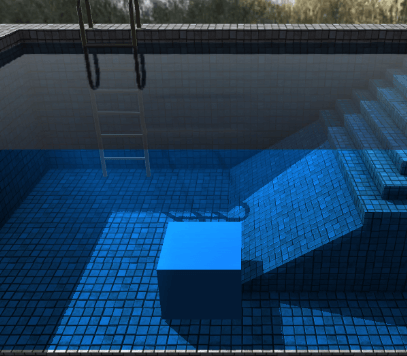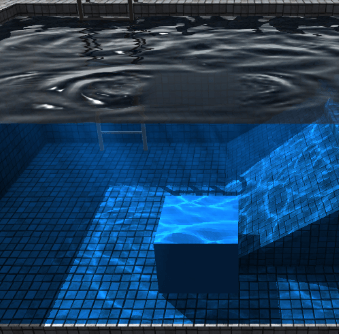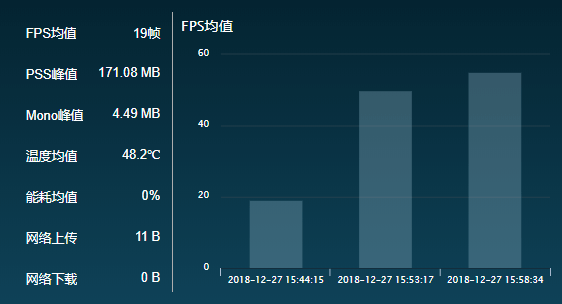• 开启全部效果时FPS均值19帧
• 关闭体积光后FPS均值50帧
• 关闭体积光关闭焦散后FPS均值55帧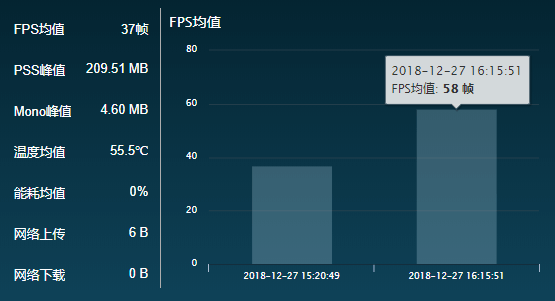• 开启全部效果时FPS均值37帧
• 不开启体积光、开启焦散，FPS均值58帧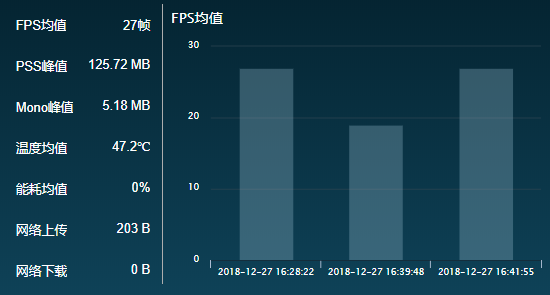• 关闭多线程渲染、不开启体积光、开启焦散FPS均值27帧
• 开启多线程渲染后，开启全部效果FPS均值19帧
• 开启多线程渲染后，关闭体积光FPS均值27帧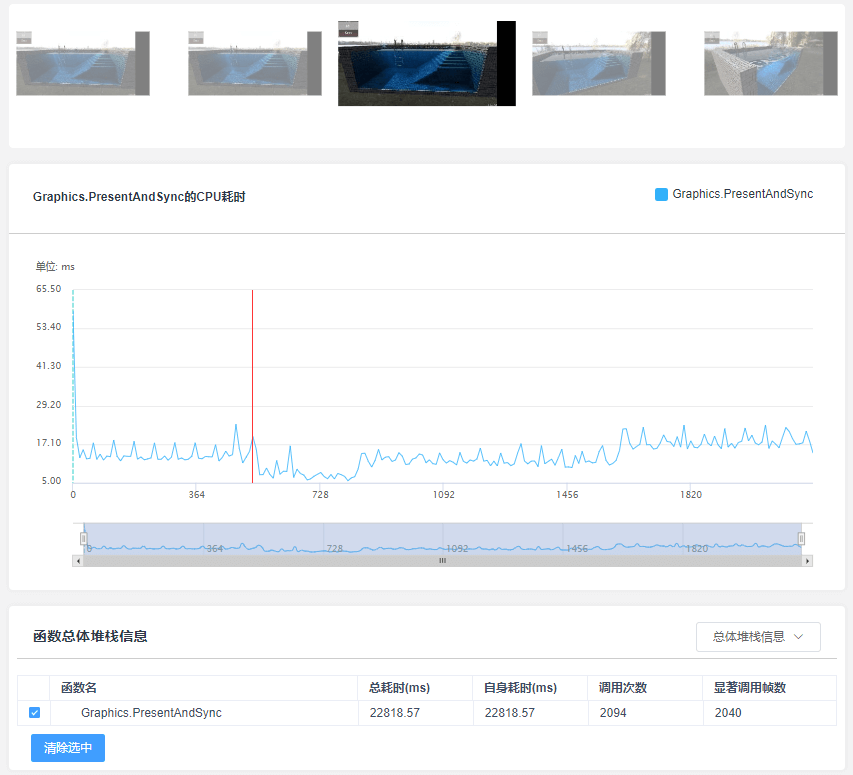Graphics.PresentAndSync在此处较高，说明当前设备上的GPU端性能压力较大，致使CPU端传输数据等待时间较长。所以，建议大家慎重选择在中低端配置的机器运行时是否要开启体积光效果。【博物纳新】是UWA旨在为开发者推荐新颖、易用、有趣的开源项目，帮助大家在项目研发之余发现世界上的热门项目、前沿技术或者令人惊叹的视觉效果，并探索将其应用到自己项目的可行性。很多时候，我们并不知道自己想要什么，直到某一天我们遇到了它。